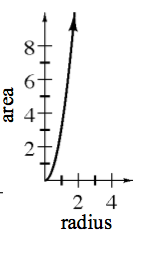### Home > CCA2 > Chapter Ch1 > Lesson 1.2.1 > Problem1-73

1-73.

Consider circles of different sizes. Create multiple representations of the function ($x→y$ table, equation, and graph) with inputs that are the radius of the circle and outputs that are its area.

How do you find the area of a circle? (If you forgot, try searching for the formula on the Internet.)

Which variable is independent, the radius or the area?

 $x$ $0$ $1$ $2$ $3$ $4$ $y$ $0$ $\pi$ $4\pi$ $9\pi$ $16\pi$